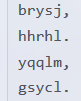(扯谈扯不下去了…….)

$$f[i]=f[j]+{|sum[i]-sum[j]-L-1|}^P$$

### Code:

#include<cmath>
#include<cstdio>
#include<string>
#include<cstring>
#include<iostream>
#include<algorithm>

typedef long long ll;
typedef long double ld;

const int NS=1e5+2;
const int inf=1e9+9;

int T,N,L,P;
int last[NS],ans[NS],Next[NS];
struct Node{int c,l,r;}q[NS];
char s[NS];
ld sum[NS],f[NS];

template <typename _Tp> inline void IN(_Tp&x){
char ch;bool flag=0;x=0;
while(ch=getchar(),!isdigit(ch))if(ch=='-')flag=1;
while(isdigit(ch))x=x*10+ch-'0',ch=getchar();
if(flag)x=-x;
}

void clear(){
memset(last,0,sizeof(last));
memset(sum,0,sizeof(sum));
memset(q,0,sizeof(q));
memset(s,0,sizeof(s));
memset(f,0,sizeof(f));
}

ld pows(ld x,int y){//快速幂
ld ans=1;
for(;y;y>>=1,x*=x)if(y&1)ans*=x;
return ans;
}

ld val(int j,int i){//转移函数
return f[j]+pows(abs(sum[i]-sum[j]-L-1),P);
}

void half(int i){//二分过程
int now=q[tail].c,ls=q[tail].l,rs=q[tail].r;//当前队尾范围
int ret=q[tail].r+1;
while(ls<=rs){
int mid=(ls+rs)>>1;
if(val(i,mid)<=val(now,mid))rs=mid-1,ret=mid;//i 更优
else ls=mid+1;//c 更优
}
if(ret!=q[tail].l)q[tail].r=ret-1;//分成了两半
else --tail;//整个元素都比不过 i
if(ret<=N)q[++tail]=(Node){i,ret,N};//i 分了一个区间时，加入新元素
}

void output(){//值得拥有的鬼畜输出
if(f[N]>1e18)puts("Too hard to arrange");//无解，放心判 1e18
else{
printf("%lld\n",(ll)(f[N]+0.5));//注意精度问题
for(int i=N;i;i=last[i])Next[last[i]]=i;//输出
int now=0;
for(int i=1;i<=N;++i){
now=Next[now];
for(int j=i;j<now;++j)printf("%s ",s[j]);
printf("%s\n",s[now]);
i=now;
}
}
puts("--------------------");//注意
return;
}

int main(){
IN(T);
while(T--){
clear();
IN(N),IN(L),IN(P);
for(int i=1;i<=N;++i){
scanf("%s",s[i]);
sum[i]=sum[i-1]+strlen(s[i])+1;//做前缀和
/*因为输出是有空格的，所以加上一个 1*/
}
for(int i=1;i<=N;++i){
half(i);//二分
}
output();//鬼畜输出
}
return 0;//终于结束
}$QvQ$ 有毒啊……

QAQ

### 2 条评论#### quhengyi11 · 2019年2月25日 9:57 下午

qwq 问个文题无关的问题

luogu 的 material 化不是自从换了主页之后咕掉了喵